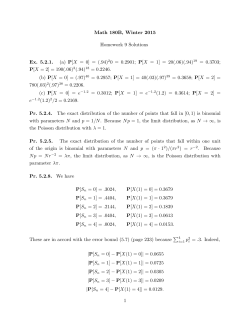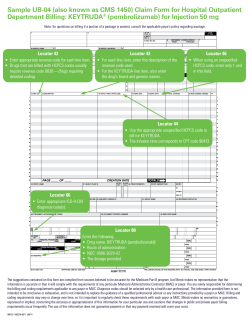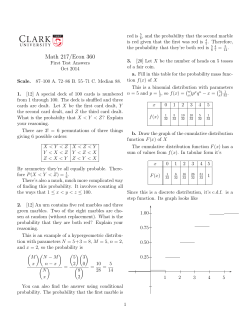# Exercise: 8 Q1. Let X and X be independent random variables, X

```Exercise: 8
Q1. Let X1 and X2 be independent random variables, Xi = B(ni, p) i= 1, 2 . Use m.g.f’s to find the
distribution of X1 - X2 + n2.
Q2. Let (X1, X2) be a bivariate normal random variables with parameters µ1, µ2 , σ12 , σ22 and ρ.
Show, using m.g.f’s, that Y and Z , given by Y=X1 - µ1 , Z= X - µ2 - ρ. σ2 (X1-µ1) / σ1 are independent
random variables and that Y and Z are each normal random variables.
Q3. Let X and Y be independent random variables each N(0,1), Show that XY is X12.
Q4. From an urn containing 10 identical balls numbered 0, 1,…..,9, n balls are drawn with
replacement. In the following let occurrence of 0 on a draw mean that the draw yields a ball with
number 0.
(a) What does the Weak Law of Large Numbers assert about the occurrence of 0’s in n drawings?
(b) How many drawing must be made in order that, with probability at least 0.95, the relative
frequency of occurrence of 0’s will be between 0.99 and 0.11?
(c) Use the Central Limit Theorem to find the probability that among n numbers thus chosen 0
will appear between
n+3 n
n−3 n
and
times.
10
10
[Given Ф(0.3) = 0.6179, Ф(0.7) = 0.7580, Ф(1.0) = 0.8413]
Q5. An employee in a call center works form 8 a.m. until 5 p.m., with breaks between 10.30-10.45,
12.30-13.30 and 14.45-15.00. Assume that calls come in according to a Poisson process with expected
number of calls per hour equal to 6.
(a) What is the probability that there are at most 10 calls during the breaks?
(b) What is the probability that first call of the day is after 8.10 a.m.?
(c) What is the probability that the employee can do something else for 45 minutes without being
disturbed by a call?
Q6. Consider a Poisson process with parameter λ. What is the conditional probability that N(1) = n
given that N(3) = n? Do you understand why this probability does not depend on λ ?
Q7. One can compute the exact distribution of XN(t)+1, the time interval between the last occurrence
before time t and the first occurrence after time t. Show that the density f of XN(t)+1 is given by
λ2 xe − λx , for , x < t ,
f ( x) = 
λ (1 + λt )e −λx , for , x < t ,
and compute the expectation of XN(t)+1 using this density.
Q8. Suppose you arrive at a single teller bank to find four other customers in the bank, one being
served and the other four waiting in line. You join the end of the line. If the service times are all
exponential with rate µ, What is the expected time you will spend in the bank?
Q9. Cars pass a certain street location according to a Poisson process with rate λ. A women who
wants to cross the street at that location waits until she can see that no cars willl come by in the next
T time units.
(a). Find the probability that her waiting time is 0.
(b). Find her expected waiting time.
Q10. Consider n components with independent lifetimes which are such that component i functions
for an exponential time with rate λI . Suppose that all components are initially in use and remain so
until they fail.
(a). Find the probability that component 1 is the 2nd component to fail.
(b). Find the expected time of the 2nd failure.
```# 30 Years` Global Scale Mapping of surface Water Dynamics at 30 m# Math 180B, Winter 2015 Homework 9 Solutions Ex. 5.2.1. (a) P[X = 0# Some activities to do with your child: Indoor helping activities# Sample UB-04 (also known as CMS 1450) Claim Form for... Department Billing: KEYTRUDA (pembrolizumab) for Injection 50 mg